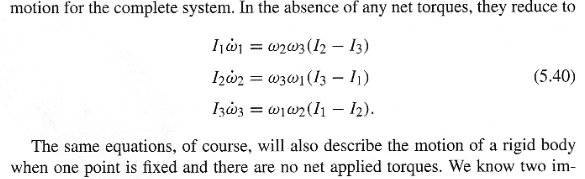# Example of torque-free rotation with a fixed point

What is an example of a rigid body rotating when one point is fixed and there are no net applied torques? And the fixed point is not the center of mass.I considered a cone rolling without slipping on a flat plane is such an example; the apex is the fixed point, but is there a net applied torque? I think there is.

Last edited:

Simon Bridge
Homework Helper
The usual bike weel suspended off its com and precessing... there is no net torque because no net angular acceleration. Same with a tilted gyroscope. Though... suffers similar analysis issues as the cone.

... I need my whiteboard to check and Im stuck in hospital (Im fine).
(I imagine it is easy with lagrangian mechanics.)
But consider, if it completes a circle, then it has returned to its initial state (its trivial to rig), so the net work is zero. So I'll say there is no unbalanced torque... but may change my mind later.

___ where Im up to ____________
For analysis... a simplified verion is: a disk of unit circumference on one end of an axle... the other end of the axle is fixed to a pivot - the whole rests on a horizontal table. The length of the axel is such that the disk is constrained to a circle with N units circumference, must stay perpendicular to the axle which is so thin and light it has zero moment of inertia. The disk rolls on its edge without slipping and has non zero initial angular velocity. Sound good?

This means that the disk rotates exactly N times when it completes one circle... so initial and final states are totally the same (after one circuit, if angular velocity is constant) so no net work was done (no non conservative forces present).

Last edited:
Simon Bridge